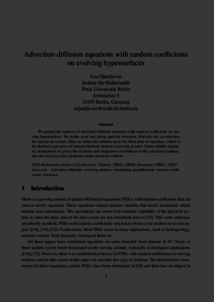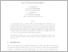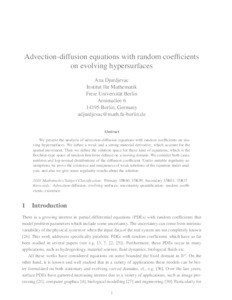Repository: Freie Universität Berlin, Math Department

# Advection-diffusion equations with random coefficients on evolving hypersurfaces

Djurdjevac, A. (2017) Advection-diffusion equations with random coefficients on evolving hypersurfaces. Interfaces and Free Boundaries, 19 (4). pp. 525-552. ISSN 1463-9963Preview

229kBPreview
PDF - Submitted Version
281kB

Official URL: https://dx.doi.org/10.4171/IFB/391

## Abstract

We present the analysis of advection-diffusion equations with random coefficients on moving hypersurfaces. We define weak and strong material derivative, that take into account also the spacial movement. Then we define the solution space for these kind of equations, which is the Bochner-type space of random functions defined on moving domain. Under suitable regularity assumptions we prove the existence and uniqueness of solutions of the concerned equation, and also we give some regularity results about the solution.

Item Type: Article 12/2015 SFB 1114 Preprint Advection-diffusion, evolving surfaces, uncertainty quantification, random coefficients, existence Mathematical and Computer Sciences > Mathematics > Applied Mathematics Department of Mathematics and Computer Science > Institute of Mathematics 1763 Ulrike Eickers 04 Jan 2016 15:36 13 Dec 2019 10:03

Repository Staff Only: item control page